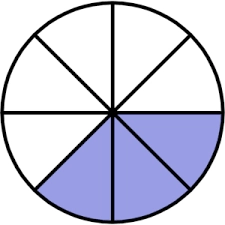# The sum

If you add 3/4 and 5/8, what would be the sum?
A.more than one
B.equal to one
C.less than one
D. zero

x = A

### Step-by-step explanation:Did you find an error or inaccuracy? Feel free to write us. Thank you!

Tips for related online calculators
Need help calculating sum, simplifying, or multiplying fractions? Try our fraction calculator.